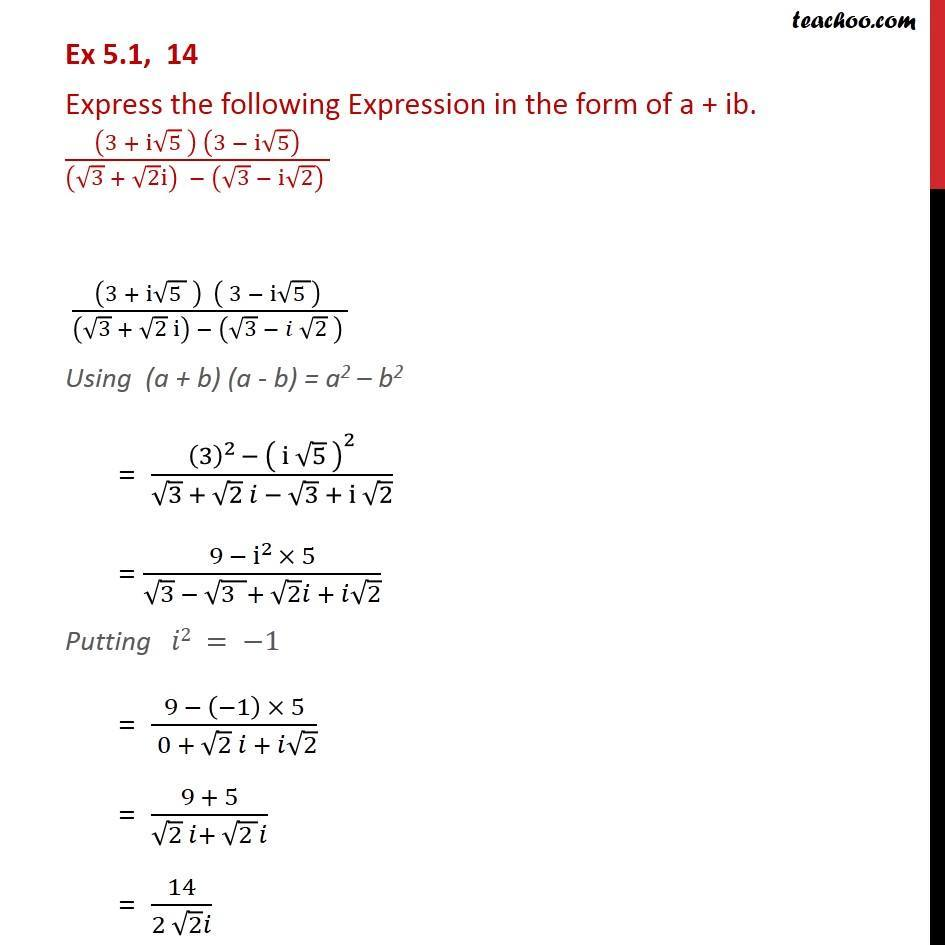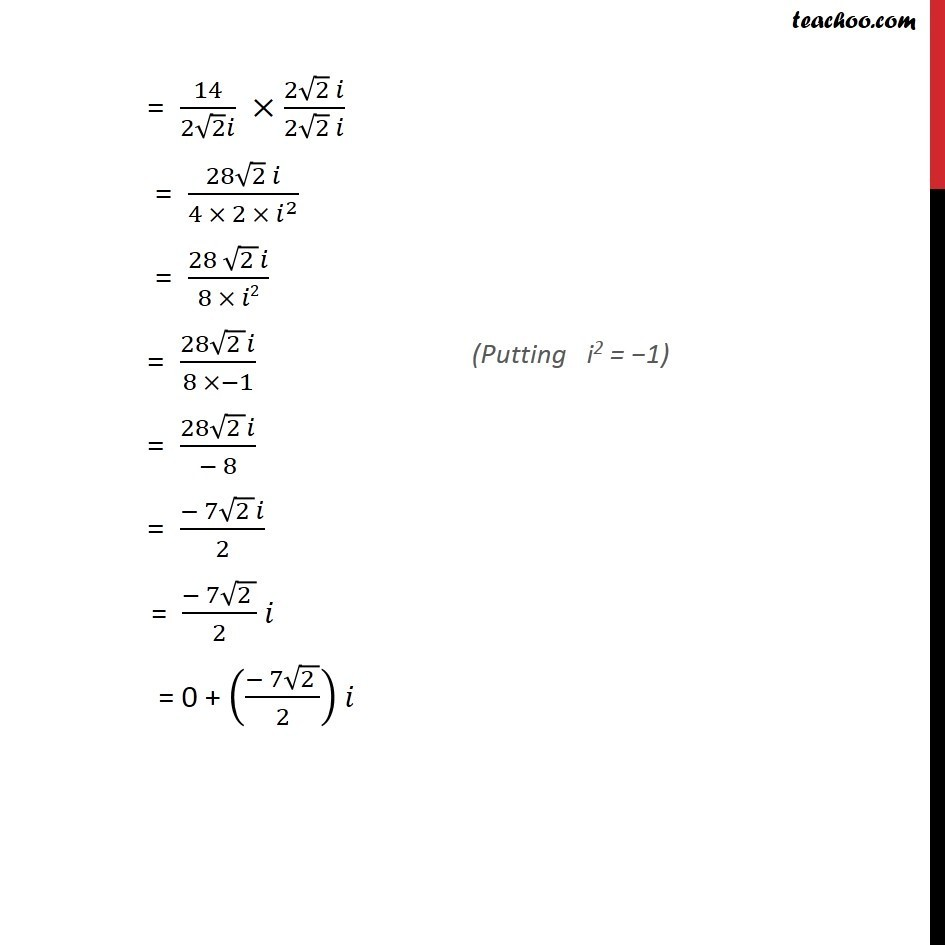1. Chapter 5 Class 11 Complex Numbers (Term 1)
2. Serial order wise
3. Ex 5.1

Transcript

Ex 5.1, 14 Express the following Expression in the form of a + ib. ((3 + i√5 ) (3 − i√5))/((√3 + √2 i) − (√3 − i√2) ) ((3 + i√(5 ) ) ( 3 − i√(5 )) )/((√3 + √2 i) − (√3 − 𝑖 √2 ) ) Using (a + b) (a - b) = a2 – b2 = ((3)^2 − ( i √5 )^2)/(√3 + √2 𝑖 − √3 + i √2) = (9 − i^2 × 5)/(√3 − √(3 )+ √2 𝑖 + 𝑖√2) Putting 𝑖2 = −1 = (9 − (−1) × 5)/( 0 + √2 𝑖 + 𝑖√2) = (9 + 5)/(√2 𝑖+ √(2 ) 𝑖) = 14/(2 √2 𝑖) = 14/(2√2 𝑖) ×(2√2 𝑖)/(2√2 𝑖) = (28√2 𝑖)/(4 × 2 × 𝑖^2 ) = (28 √(2 ) 𝑖)/(8 × 𝑖2) = (28√(2 ) 𝑖)/(8 ×−1) = (28√(2 ) 𝑖)/(− 8) = (− 7√(2 ) 𝑖)/2 = (− 7√(2 ))/2 𝑖 = 0 + ((− 7√(2 ))/2) 𝑖